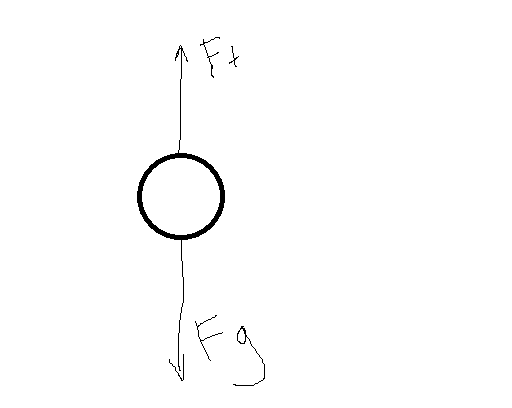# Force of Tension Question

physicsnobrain

## Homework Statement

A bowling ball is suspended from a ceiling hook using a thin piece of rope. The rope is
exerting a 75 N force upward on the ball. Suppose that the rope is unhooked from the ceiling and pulled upward so that the
bowling ball rises 1.2 meters in 1.5 seconds. What will be the tension in the rope during
this time?

No clue

## The Attempt at a Solution

I'm completely lost.

Homework Helper

## Homework Statement

A bowling ball is suspended from a ceiling hook using a thin piece of rope. The rope is
exerting a 75 N force upward on the ball. Suppose that the rope is unhooked from the ceiling and pulled upward so that the
bowling ball rises 1.2 meters in 1.5 seconds. What will be the tension in the rope during
this time?

No clue

## The Attempt at a Solution

I'm completely lost.
Can you draw a vector diagram showing all the forces on the ball? What must these force vectors sum to?

AM

physicsnobrain
Can you draw a vector diagram showing all the forces on the ball? What must these force vectors sum to?

AMI can find the Vy by doing v=d/t, then using the equation y = yi + yvit + 1/2at^2 to find the acceleration. I find the y velocity to be 0.8 m/s and the acceleration to be 1.06667 m/s^2. After this I have no idea waht to do

physicsnobrain
Is this question possible to solve? Does anyone know?

physicsnobrain
Huzzah! I have figured it out!!!!!

First one must solve for the velocity in the y direction by doing v=d/t which is 1.2m/1.5s and this equals to 0.8m/s.

Now since you have the velocity you can now solve for the acceleration.

We use this equation:

y = Yi + Yvit + 1/2at^2

Solving for acceleration we get: 1.06667 m/s^2

Now since we have the acceleration one can now solve for the mass using

F = ma

75N = m(1.06667)

m= 70.3 kg

Now since we have the mass we can solve for force of tension using this

Fnet = Ft - Fg

we use this because we have to do Ft - Fg because Ft is bigger than Fg obviously because if it wasn't then gravity would pull it down but this is not the case since it is moving up.

Anyways, since Fnet = ma we will solve for Ft (the force of tension or simply put tension) because this is what the question asks us to do !!!

Anyways now we can rearrange that formula and turn it into this to solve for tension because we are looking for it:

Ft = Fnet + Fg

which equals:

Ft = ma + mg
Ft = 70.3kg(1.06667) + (70.3kg)(9.81)

Ft = 764 N

The force of tension is 764N.

Thanks for all the help and information everyone!! It was sooo useful!!

Homework Helper
Huzzah! I have figured it out!!!!!

First one must solve for the velocity in the y direction by doing v=d/t which is 1.2m/1.5s and this equals to 0.8m/s.

Now since you have the velocity you can now solve for the acceleration.

We use this equation:

y = Yi + Yvit + 1/2at^2

Solving for acceleration we get: 1.06667 m/s^2

Now since we have the acceleration one can now solve for the mass using

F = ma

75N = m(1.06667)

m= 70.3 kg

Now since we have the mass we can solve for force of tension using this

Fnet = Ft - Fg

we use this because we have to do Ft - Fg because Ft is bigger than Fg obviously because if it wasn't then gravity would pull it down but this is not the case since it is moving up.

Anyways, since Fnet = ma we will solve for Ft (the force of tension or simply put tension) because this is what the question asks us to do !!!

Anyways now we can rearrange that formula and turn it into this to solve for tension because we are looking for it:

Ft = Fnet + Fg

which equals:

Ft = ma + mg
Ft = 70.3kg(1.06667) + (70.3kg)(9.81)

Ft = 764 N

The force of tension is 764N.

Thanks for all the help and information everyone!! It was sooo useful!!
The only problem is that it is not correct! The mass of the ball is nowhere near 70.3 kg.!! No one could lift it if that were the case.

The force of tension is 75N when the ball is stationary. So what does that tell you about the mass of the ball?

Once you have m, you can use your equation:

Ft = mg + ma

AM